### Home > CALC > Chapter 6 > Lesson 6.2.2 > Problem6-78

6-78.
1. For each of the following functions, find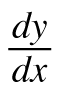. Homework Help ✎

1.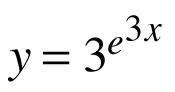2.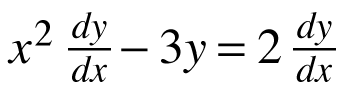3.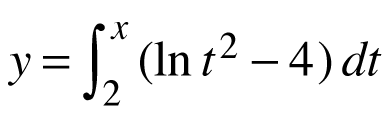4.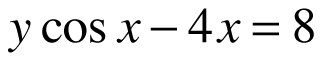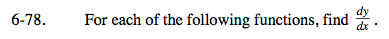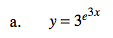Use the Chain Rule. You may have to use it more than once.

The derivative of ax is (ln a) · ax. For ex, due to the nature of ln, its derivative is itself.

$\text{Use algebra to solve for }\frac{dy}{dx}.$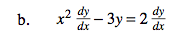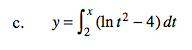What is the derivative of an integral?
Also, should your result be in terms of t or in terms of x?
To answer this, imagine the result of actually integrating the function first before differentiating it.

$y'=\frac{4+y\text{sin}x}{\text{cos}x}$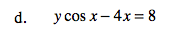Use implicit differentiation.